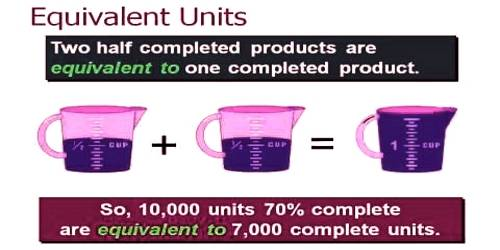# Equivalent Production Unit

Equivalent Production Unit

An equivalent unit of production is an indication of the amount of work done by manufacturers who have partially completed units on hand at the end of an accounting period. It is a term applied to the work-in-process inventory at the end of an accounting period. Basically, the fully completed units and the partially completed units are expressed in terms of fully completed units. It is a cost accounting concept that is used in process costing for cost calculations.

Equivalent units must be considered relative to each of the factors of production. In other words, 80% of necessary direct material may be in process but only 60% of the direct labor and factory overhead. Therefore, proper costing methodology for 100 units in the process would entail 80 equivalent units of material, and 60 equivalent units of conversion (i.e., labor and overhead).

To assess the equivalent units of production requires careful reasoning about the amount of direct material injected into production for each department, relative to the total amount of direct material that will ultimately be needed to complete the process within that department. This type of assessment must be repeated for direct labor and overhead. If overhead is applied based on labor, the process is simplified because the “percent complete” would be the same for labor and overhead.

However, if overhead were applied on some other basis (like machine hours) then a separate determination of equivalency would be needed for labor and for overhead (the illustrations within this chapter will assume overhead is applied based on labor).